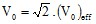# Describing sources

## Introduction

The only one possible type of source is characterized by:

• an imposed value of the electric harmonic potential (V0)

The Flux describing of this type of source is detailed in the following blocks.

## Imposed electric potential

It is possible to impose an electric harmonic potential (V0) on a region by using the regions known as non-material of the type: imposed electric potential.

The electric potential imposed on a face region (3D) or on a line region (2D/3D) is a time harmonic function:

V(t) = V0ejωt withIt is defined by:

• the rms value (in V)
• the phase (in degrees)

These two values can be uniform or space dependent.